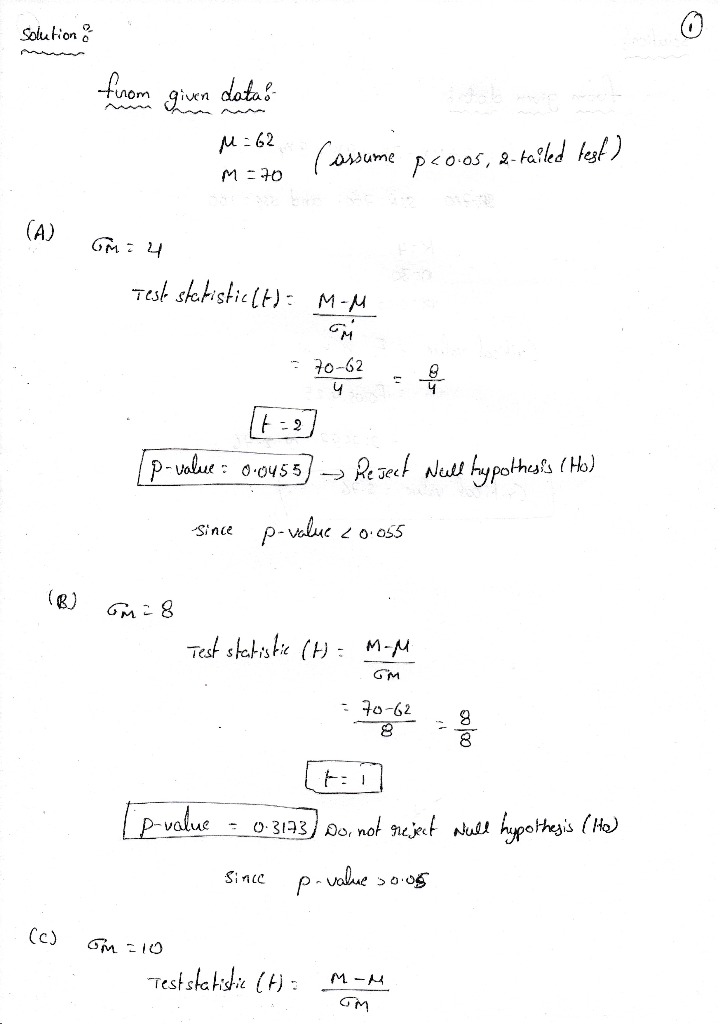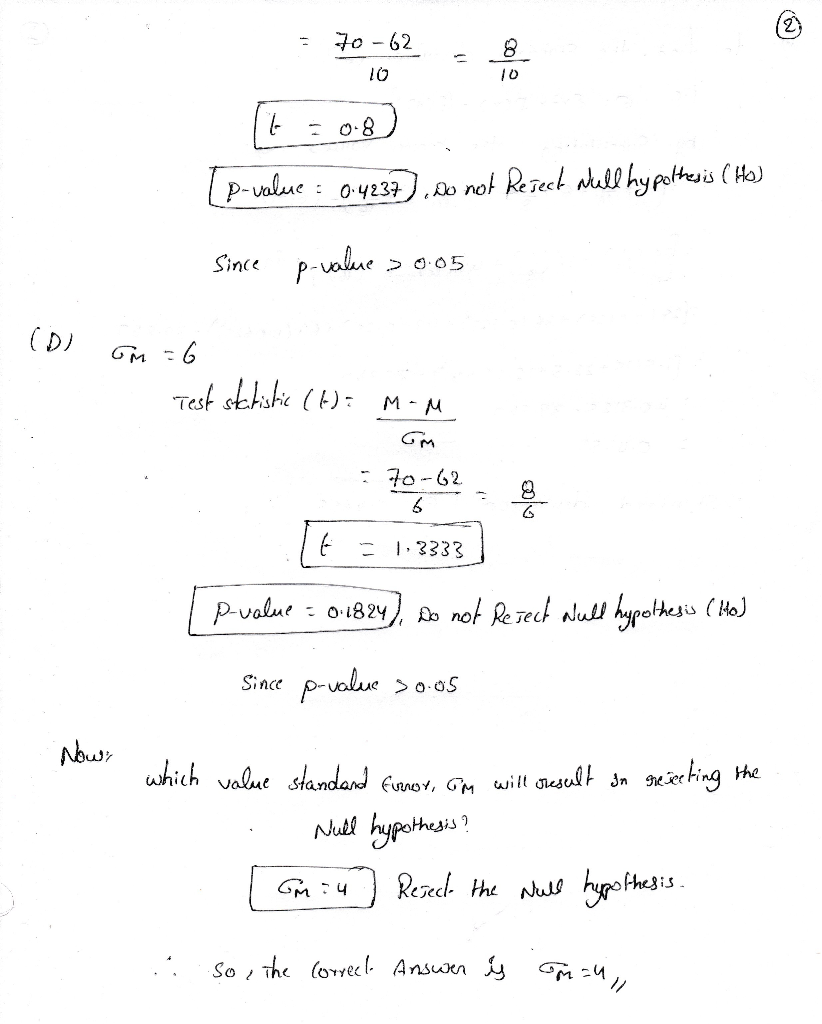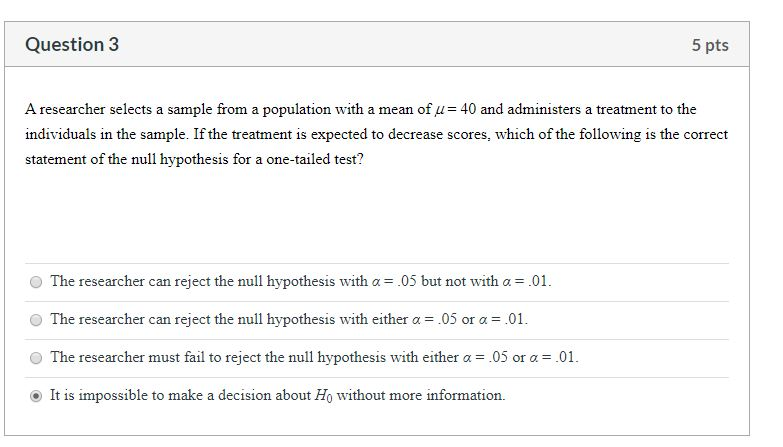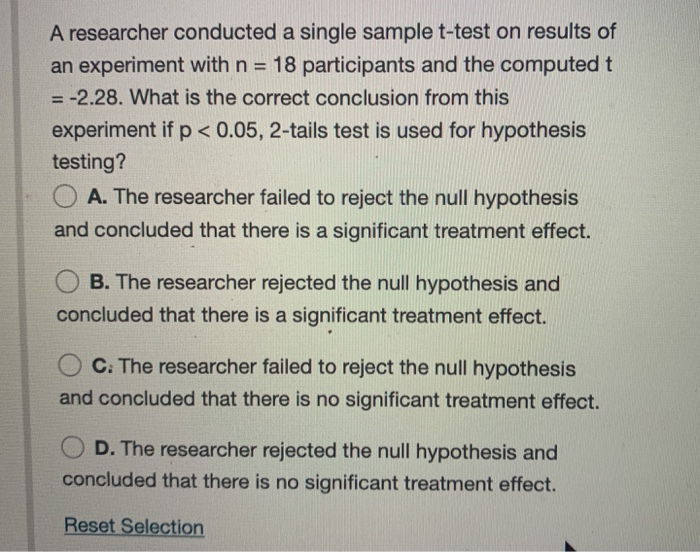Question

# A researcher administers a treatment to a sample of participants selected from a population with µ...

A researcher administers a treatment to a sample of participants selected from a population with µ = 62. If the sample mean after the treatment is M = 70, which value of standard error,σM will result in the rejection of null hypothesis (assume p < 0.05, 2-tails test)?

• A. σM = 4

• B. σM = 8

• C. σM = 10

• D. σM = 6

Please explain each step in detail, thank you!#### Earn Coins

Coins can be redeemed for fabulous gifts.

Similar Homework Help Questions
• ### A random sample of n = 12 individuals is selected from a population with µ =...

A random sample of n = 12 individuals is selected from a population with µ = 70, and a treatment is administered to each individual in the sample. After treatment, the sample mean is found to be M = 74.5 with SS = 297. Use the Distributions tool to help answer the questions that follow. t Distribution Degrees of Freedom = 21 -3.0-2.0-1.00.01.02.03.0x.5000.50000.000 QUESTION: How much difference is there between the mean for the treated sample and the mean for...

• ### --------A sample of 36 observations is selected from a normal population. The sample mean is 34,...

--------A sample of 36 observations is selected from a normal population. The sample mean is 34, and the population standard deviation is 5. Carry out a hypothesis test (with a level of significance α of 0.05) of the null hypothesis H0: µ ≥ 35 using the 6-step procedure ---------Suppose that someone claims that the mean number of sick days taken by U.S. employees is 5.1. You decide to investigate that claim and take a representative sample of 87 U.S. employees...

• ### Question 3 5 pts A researcher selects a sample from a population with a mean of...Question 3 5 pts A researcher selects a sample from a population with a mean of u= 40 and administers a treatment to the individuals in the sample. If the treatment is expected to decrease scores, which of the following is the correct statement of the null hypothesis for a one-tailed test? The researcher can reject the null hypothesis with a = .05 but not with a = .01. The researcher can reject the null hypothesis with either a =...

• ### PLEASE help ?? A researcher conducted a single sample t-test on results of an experiment with...PLEASE help ?? A researcher conducted a single sample t-test on results of an experiment with n = 18 participants and the computed t = -2.28. What is the correct conclusion from this experiment if p < 0.05, 2-tails test is used for hypothesis testing? O A. The researcher failed to reject the null hypothesis and concluded that there is a significant treatment effect. O B. The researcher rejected the null hypothesis and concluded that there is a significant treatment...

• ### A sample of n = 6 individuals is selected from a population with µ = 25....

A sample of n = 6 individuals is selected from a population with µ = 25. After a treatment is administered to the individuals, the sample mean is found to be M = 27. A. If the sample variance is s = 4, then conduct a hypothesis test to evaluate the significance of the treatment effect and calculate r2 to measure the size of the treatment effect. Use a two-tailed test with α = .05. B. If the sample variance...

• ### Assume that a simple random sample has been selected from a normally distributed population and test...

Assume that a simple random sample has been selected from a normally distributed population and test the given claim. Use either the traditional method or P-value method as indicated. Identify the null and alternative hypotheses, test statistic, critical value(s) or P-value (or range of P-values) as appropriate, and state the final conclusion that addresses the original claim. -------------------------------- QUESTION: Use a significance level of ΅ = 0.05 to test the claim that µ = 32.6. The sample data consist of...

• ### A normally distributed population has a mean of µ = 70 and a standard deviation of...

A normally distributed population has a mean of µ = 70 and a standard deviation of σ = 12. A sample (n = 36) is selected from a population and a treatment is administered to the sample. After treatment, the sample mean is found to be M = 65.  Does this sample provide evidence of a statistically significant treatment effect with an alpha of 0.05 (non-directional hypothesis)?  [G&W Chp 8] Yes, our z-score reaches the critical region. No, our z-score fails to...

• ### 4. It is desired to test H0 : µ = 20 Vs H1 : µ <...

4. It is desired to test H0 : µ = 20 Vs H1 : µ < 20, on the basis of a random sample of size 64 from normal distribution with population standard deviation σ = 2.4. The sample mean and sample standard deviation are found to be 19.5 and 2.5, respectively. (a) Test the hypothesis at α = 0.05. Compute the test statistics, critial regions, and perform the test. Will the result be difference if α is changed to...

• ### A sample of n = 16 individuals is selected from a population with µ = 40...

A sample of n = 16 individuals is selected from a population with µ = 40 and σ = 12, and a treatment is administered to the sample. After treatment, the sample mean is M = 42. You are asked to determine if the sample is still the same as the population. Using an alpha of .05 and a directional (one-tailed) hypothesis because you expect an increase in your sample mean due to the treatment, conduct a one-sample z-test and...

• ### To test the effectiveness of a treatment, a sample of n = 36 people is selected...

To test the effectiveness of a treatment, a sample of n = 36 people is selected from a normal population with mean of μ = 60. After the treatment is administered to the individuals in the sample, the sample mean is found to be M = 55. (a) If the population standard deviation is σ = 13, can you conclude that the treatment has a significant effect? Use a two-tailed test with α = 0.05. (Round your answers to two...